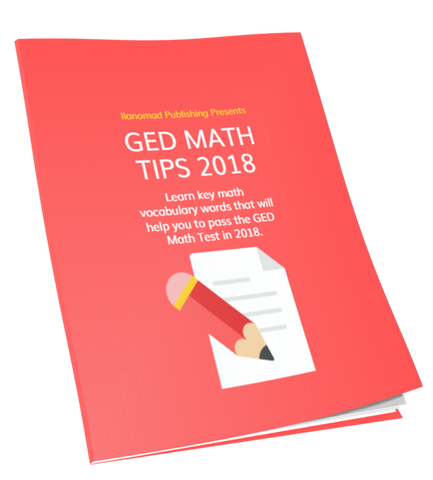# GED Math Tips 2018

Even though the passing mark has been reduced to 145 from 150 and the time to take the GED Math Test has been extended to 115 minutes, most students still struggle to pass the GED Math Test.

Because of this reality, every point is important.

I've created GED Math Tips 2018 to give you an advantage and help you to earn a few more points that may help put you over the top!

GED Math Vocabulary

On the GED Test, many mathematical terms are used in the questions. For example, do you know the difference between an expression and an equation? If you don't, it's a good idea to know the difference before you take the GED Math Test.

GED Math Tips 2018 will help you gain more points on the GED Math Test by teaching you three key math vocabulary words. In this eBook, I actually show you screenshots from an Official GED Math Practice Test that features questions involving math vocabulary.

It is pretty simple, if you don't know what these terms mean, you probably will not be able to answer these question types correctly.

On the flip-side, having these GED Math Tips ahead of time will help you to be able to answer these questions correctly. That's how GED Math Tips 2018  will help you get more points on the GED Math Test.